Homework Help Question & Answers

# Independent random samples were selected from two quantitative populations, with sample sizes, means, and standard deviations...

Independent random samples were selected from two quantitative populations, with sample sizes, means, and standard deviations given below.
n1= 55, n2= 65, xbar1= 35.5, xbar2= 31.4, s1= 5.7, s2= 3.3
1.) Construct a 95% confidence interval for the difference in the population means (mu1- mu2). (Round your answers to two decimal places)
2.) Find a point estimate for the fifference in the population means.
3.) Calculate a margin of error. (Round your answer to two decimal places)

#### Homework Answers

Answer #1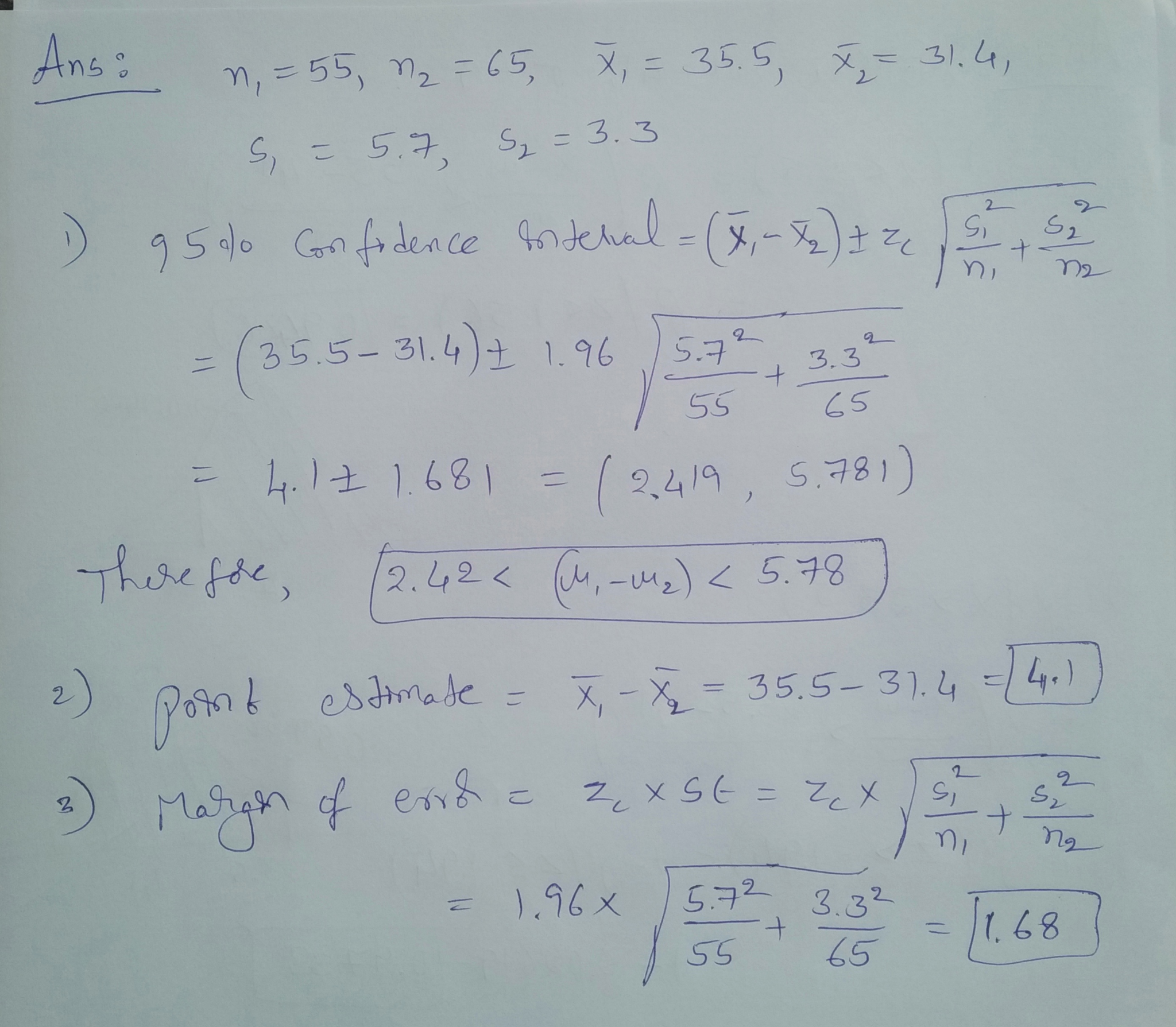Know the answer?
Your Answer:

#### Post as a guest

Your Name:

What's your source?

#### Earn Coin

Coins can be redeemed for fabulous gifts.

Not the answer you're looking for? Ask your own homework help question. Our experts will answer your question WITHIN MINUTES for Free.
Similar Homework Help Questions
• ### Independent random samples were selected from two quantitative populations, with sample sizes, means, and standard deviations...

Independent random samples were selected from two quantitative populations, with sample sizes, means, and standard deviations given below. n1 = n2 = 60 x1 = 125.3 x2 = 123.4 s1 = 5.7 s2 = 6.1 a) Construct a 95% confidence interval for the difference in the population means (μ1 − μ2). (Round your answers to two decimal places.) to b) Find a point estimate for the difference in the population means. c) Calculate the margin of error. (Round your answer...

• ### Independent random samples were selected from two quantitative populations, with sample sizes, means, and standard deviations...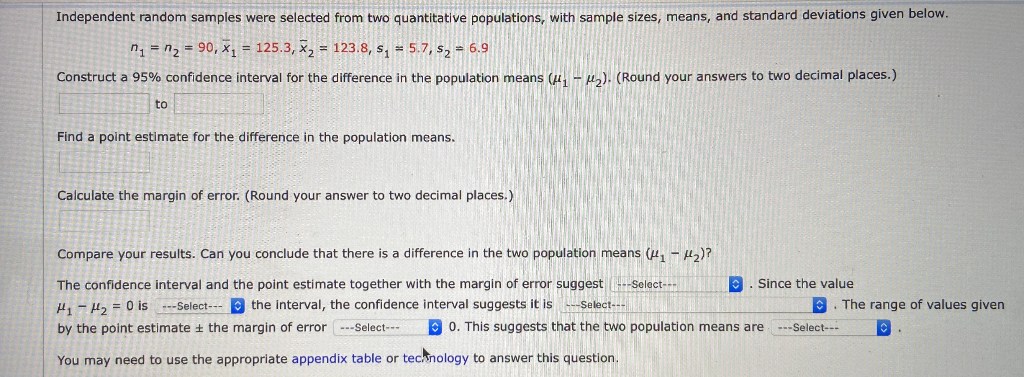Independent random samples were selected from two quantitative populations, with sample sizes, means, and standard deviations given below. n = n2 = 90, x1 = 125.3, %2 = 123.8, s, = 5.7, s, = 6.9 Construct a 95% confidence interval for the difference in the population means ( M M ) (Round your answers to two decimal places.) Find a point estimate for the difference in the population means, Calculate the margin of error. (Round your answer to two decimal...

• ### Two random samples are selected from two independent populations. A summary of the samples sizes, sample...

Two random samples are selected from two independent populations. A summary of the samples sizes, sample means, and sample standard deviations is given below: n1=51, n2=46, x¯1=57.8, x¯2=75.3, s1=5.2 s2=11 Find a 94.5% confidence interval for the difference μ1−μ2μ1−μ2 of the means, assuming equal population variances. Confidence Interval =

• ### Independent random samples selected from two normal populations produced the sample means and standard deviations shown...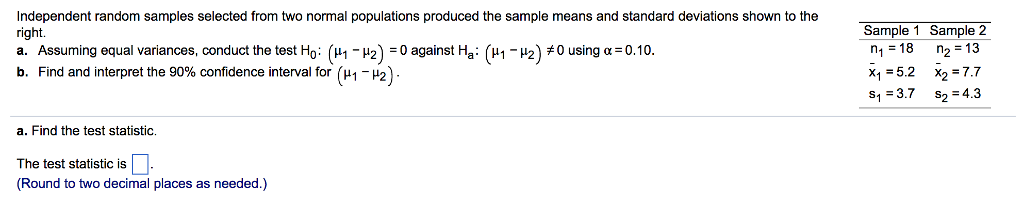Independent random samples selected from two normal populations produced the sample means and standard deviations shown to the right. a. Assuming equal variances, conduct the test Ho ??-?2):0 against Ha : (??-?2)#0 using ?:010. b. Find and interpret the 90% confidence interval for ( 1- 2)- Sample 1 Sample 2 n1 18 n2 13 x1-5.2 x27.7 s1 3.7 s2 4.3 a. Find the test statistic. The test statistic is (Round to two decimal places as needed.)

• ### Independent random samples were selected from two quantitative populations, with sample sizes, means, and variances given...

Independent random samples were selected from two quantitative populations, with sample sizes, means, and variances given below. Population 1 2 Sample Size 39 44 Sample Mean 9.3 7.3 Sample Variance 8.5 14.82 Construct a 90% confidence interval for the difference in the population means. (Use μ1 − μ2. Round your answers to two decimal places.) __________ to ____________ Construct a 99% confidence interval for the difference in the population means. (Round your answers to two decimal places.) __________ to _____________

• ### Two samples are taken with the following sample means, sizes, and standard deviations

Two samples are taken with the following sample means, sizes, and standard deviations¯x1x¯1 = 33 ¯x2x¯2 = 26n1n1 = 55 n2n2 = 52s1s1 = 3 s2s2 = 4Find a 97% confidence interval, round answers to the nearest hundredth.___ < μ1−μ2μ1-μ2 < ___

• ### Two samples are taken with the following sample means, sizes, and standard deviations ¯x1 = 25...

Two samples are taken with the following sample means, sizes, and standard deviations ¯x1 = 25 ¯x2 = 23 n1 = 54 n2 = 73 s1 = 5 s2 = 3 Estimate the difference in population means using a 88% confidence level. Use a calculator, and do NOT pool the sample variances. Round answers to the nearest hundredth.

• ### Independent random samples selected from two normal populations produced the sample means and standard deviations shown...

Independent random samples selected from two normal populations produced the sample means and standard deviations shown to the right. a) Assuming equal​ variances, conduct the test Ho: (u1-u2)=0 against Ha: (u1-u2)=/=0 using a=0.05 b) Find and interpret the 95% confidence interval for (u1-u2) Sample1: n1=17, x1=5.9, s1=3.8 Sample2: n2=10, x1=7.3, s2=4.8

• ### Independent random samples were selected from two quantitative populations, with sample sizes, means, and variances given...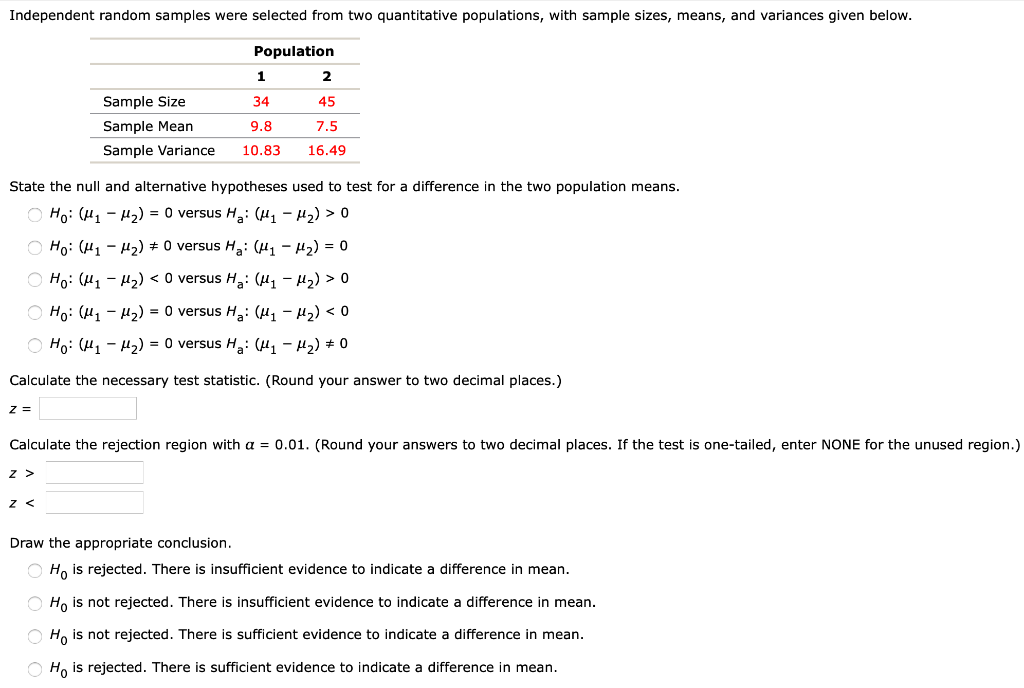Independent random samples were selected from two quantitative populations, with sample sizes, means, and variances given below. Sample Size Sample Mean Sample Variance Population 1 2 34 45 9.8 7.5 10.83 16.49 State the null and alternative hypotheses used to test for a difference in the two population means. O Ho: (41 - H2) = 0 versus Ha: (41 - M2) > 0 Ho: (41 – 12) # O versus Ha: (H1 - H2) = 0 HO: (41 – My)...

• ### = 3.0. The sample standard deviations were Samples were drawn from three populations. The sample sizes...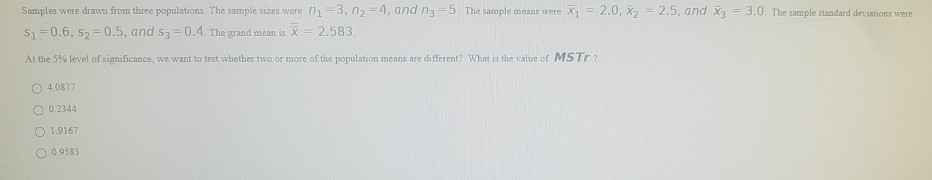= 3.0. The sample standard deviations were Samples were drawn from three populations. The sample sizes were ni =3, n2 = 4, and n3=5 The sample means were X1 = 2.0, X2 = 2.5, and x3 Si=0.6, 52=0.5, and S3 =0.4. The grand mean is x = 2.583 At the 5% level of significance, we want to test whether two or more of the population means are different? What is the value of MST? 4.0877 O 0.2344 1.9167 O 0.9583

Free Homework App

Scan Your Homework
to Get Instant Free Answers
Need Online Homework Help?

Get Answers For Free
Most questions answered within 3 hours.